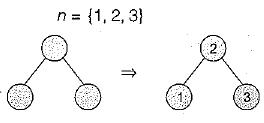Courses

# Trees (Advance Level) - 1

## 15 Questions MCQ Test Question Bank for GATE Computer Science Engineering | Trees (Advance Level) - 1

Description
This mock test of Trees (Advance Level) - 1 for Computer Science Engineering (CSE) helps you for every Computer Science Engineering (CSE) entrance exam. This contains 15 Multiple Choice Questions for Computer Science Engineering (CSE) Trees (Advance Level) - 1 (mcq) to study with solutions a complete question bank. The solved questions answers in this Trees (Advance Level) - 1 quiz give you a good mix of easy questions and tough questions. Computer Science Engineering (CSE) students definitely take this Trees (Advance Level) - 1 exercise for a better result in the exam. You can find other Trees (Advance Level) - 1 extra questions, long questions & short questions for Computer Science Engineering (CSE) on EduRev as well by searching above.
QUESTION: 1

### Level order traversal of a rooted tree can be done by starting from the root and performing

Solution:

Level order traversal of rooted tree can be done by starting from root and visiting all node at k level before visiting any node at k + 1 level, which is nothing but breadth first search.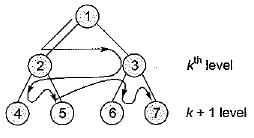QUESTION: 2

### The elements 32, 15, 20, 30, 12, 25, 16 are inserted one by one in the given order into a maxHeap. The resultant maxHeap is

Solution:

Input sequence 32,15, 20, 30,12, 25,16 If i is the root then A [i] > A [2i] for 1 < i < n/2 and A [i] > A [2i + 1] for 1 < i < n/2 where 2i is the left of i and 2i + 1 is the right of i of a max heap.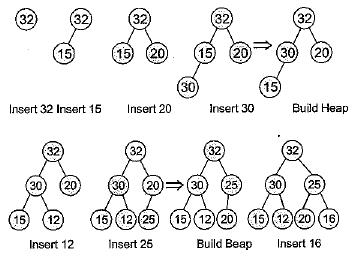QUESTION: 3

### Which one of the following binary trees has its inorder and preorder traversals as BCAD and ABCD, respectively?

Solution: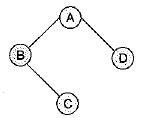Inorder traversal is BCAD and preorder traversal is ABCD.

QUESTION: 4

The numbers 1 , 2 .... n are inserted in a binary search tree in some order, In the resulting tree, the right subtree of the root contains p nodes. The first number to be inserted in the tree must be

Solution:

Number 1 ,2 , 3, ....... n are inserted into BST in some order.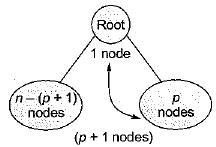Since we know total nodes = n
So, Left (root) + 1 + p = n
Left (root) = n - p - 1
Left (root) = n - (p + 1)
So, root element will be = left element + 1
= n - (p + 1) + 1
= n - p - 1 + 1
= n - p

QUESTION: 5

A binary search tree contains the numbers 1,2, 3, 4, 5, 6, 7, 8, When the tree is traversed in preorder and the values in each node printed out, the sequence of values obtained is 5, 3,1, 2, 4, 6, 8, 7. If the tree is traversed in postorder, the sequence obtained would be

Solution: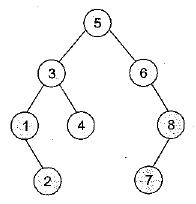QUESTION: 6

How many distinct binary search trees can be created out of 4 distinct keys?

Solution:

Total number of distinct binary search tree with n nodes (key)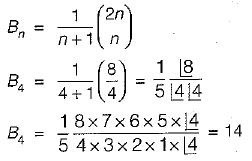QUESTION: 7

In a complete k-ary, every internal node has exactly k children. The number of leaves in such a tree with n internal nodes is

Solution:

In a complete-k-ary tree. If every internal node has exacity k children then (k - 1) key contains no leaves, if there are n internal nodes, then number of leaves is n(k- 1) + 1.

QUESTION: 8

In a binary max heap containing.n numbers, the smallest element can be found in time

Solution:

MAX Heap used to identity max element in O(1) time for to identity min element require O(n), to apply the linear search.

QUESTION: 9

In a binary tree, the number of internal nodes of degree 1 is 5, and the number of internal nodes of degree 2 is 10. The number of leaf nodes in the binary tree is

Solution:

Let number of vertices in binary tree = n Number of edges = n - 1
So, n - 1 = 1 x 5 + 2 x 10
n - 1 = 5 + 20
n = 26
Num ber of leaf nodes = 26 - 5 - 10 = 11

QUESTION: 10

The inorder and preorder traversal of a binary tree are “dbeafcg" and “abdecfg’’ respectively. The postorder traversal of the binary tree is

Solution:

The in order traversal sequence is dbeafcg and the pre order traversal sequence is abdecfg.
So the tree is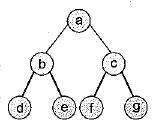In the post order traversal the sequence is debfgca.

QUESTION: 11

Consider the process of inserting an element into a Max Heap, where the Max Heap is represented by an array. Suppose we perform a binary search on the path from the new leaf to the root to find the position for the newly inserted element, the number of comparisons performed is

Solution:

After inserting an element at leaf in max heap we perform binary search on the path from the new leaf to the root to find the correct position for the newly inserted element. Comparisions will take θ(loglogn) but time will be θ(logn) because of swap operations.

QUESTION: 12

How many distinct BSTs can be constructed with 3 distinct keys?

Solution:

For distinct BST we apply this formula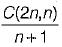n = 3 here so C(6, 3) = 20 and 20/4 = 5

QUESTION: 13

What is the maximum height of any AVL-tree with 7 nodes? Assume that the height of a tree with a single node is 0.

Solution:

Maximum height of any AVL-tree with 7.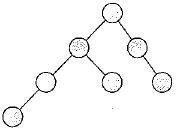(There may be different way to draw AVL with 7 nodes).

QUESTION: 14

A max-heap is a heap where the value of each parent is greater than or equal to the value of its children. Which of the following is a max-heap?

Solution: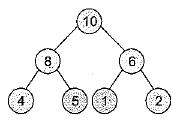QUESTION: 15

We are given a set of n distinct elements and an unlabeled binary tree with n nodes. In how many ways can we populate the tree with the given set so that it becomes a binary search tree?

Solution: